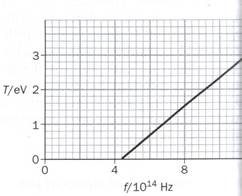CATEGORIES:

# Coursework 10 MODERN PHYSICS

Planck constant h = 6.63 × 10-34 J s = 4.14 × 10-15 eV s

The ionisation energy of a hydrogen atom is 13.6 eV

Mass of electron me = 9.11 × 10-31 kg

Speed of light = 3.00 × 108 m s-1

1) Write one paragraph to explain the photoelectric effect. In your paragraph, include a clearly marked diagram and any relevant equation(s). Use all the following words in your explanation:

absorb / emit or eject / incident light / kinetic energy / stopping voltage / work function / photoelectrons / metal / surface / threshold frequency. (8 marks)

2) The work function of sodium metal is 2.4 eV. What is the longest wavelength light that can cause electrons to be emitted from the surface of sodium?

(3 marks)

3) A beam of light from a HeNe laser with wavelength 633 nm is emitted with a power of 3.0 mW. How many photons pass a given point in the beam each second?

(3 marks)

4) UV light of wavelength 207 nm causes photoemission from a metal surface. The maximum energy of the photoelectrons is 2 eV. Find the work function of the surface. (3 marks)

5) The graph below shows how the maximum kinetic energy T of photoelectrons emitted from a metal surface varies with the frequency of the incident electromagnetic radiation. (6 marks)a) Why are no photoelectrons emitted below 4.4 × 1014 Hz?

b) Obtain the work function for the metal surface.

c) Use this experimental data to obtain a value for the Planck constant h.

6) a) Evaluate the wavelength emitted by a hydrogen atom when its excited electron falls from the n = 5 state to the n = 2 state.

b) What wavelength will the electron then emit as it makes a further transition? (4 marks)

7) In the hydrogen atom, what is the shortest wavelength of light emitted when electrons fall from excited states to the n = 2 state? (3 marks)

8) a) What is the largest wavelength of light that will ionize atoms of hydrogen in their ground state?

b) What happens if light with a smaller wavelength is incident on hydrogen atoms? (4 marks)

9) The ionisation energy for hydrogen is 13.6 eV, and the first and second excitation energies are 10.2 eV and 12.1 eV respectively.

a) Draw an energy level diagram showing the ground state and the next two energy levels for hydrogen.

b) Mark on your diagram the transition between the two of these levels that would result in the emission of radiation of the shortest wavelength. Calculate the value of this wavelength. (6 marks)

10) Consider an electron with a velocity of 106 m s-1, and a tennis ball, mass 0.15 kg, with a velocity of 40 m s-1. Calculate the de Broglie wavelength in each case. Explain why it is possible to observe the wave-nature of the electron but not that of the tennis ball. (5 marks)

11) Determine the minimum uncertainty in the position of each of the following particles if the speed is measured with an uncertainty of 0.1%.

a) An electron moving with a speed of 4 × 106 m s-1.

b) A object of mass 0.01 kg moving at a speed of 400 m s-1 (4 marks)

12) Explain the difference between emission spectra and absorption spectra. Give one example of each. (6 marks)

Date: 2015-01-12; view: 816

 <== previous page | next page ==> The French are arrogant. | Coursework 9 Mechanical waves and stationary waves 2011-12
doclecture.net - lectures - 2014-2020 year. Copyright infringement or personal data (0.002 sec.)